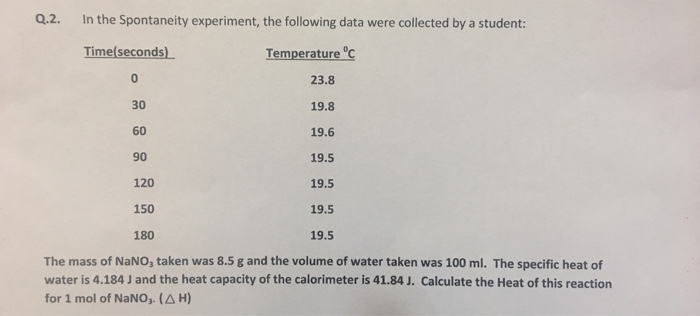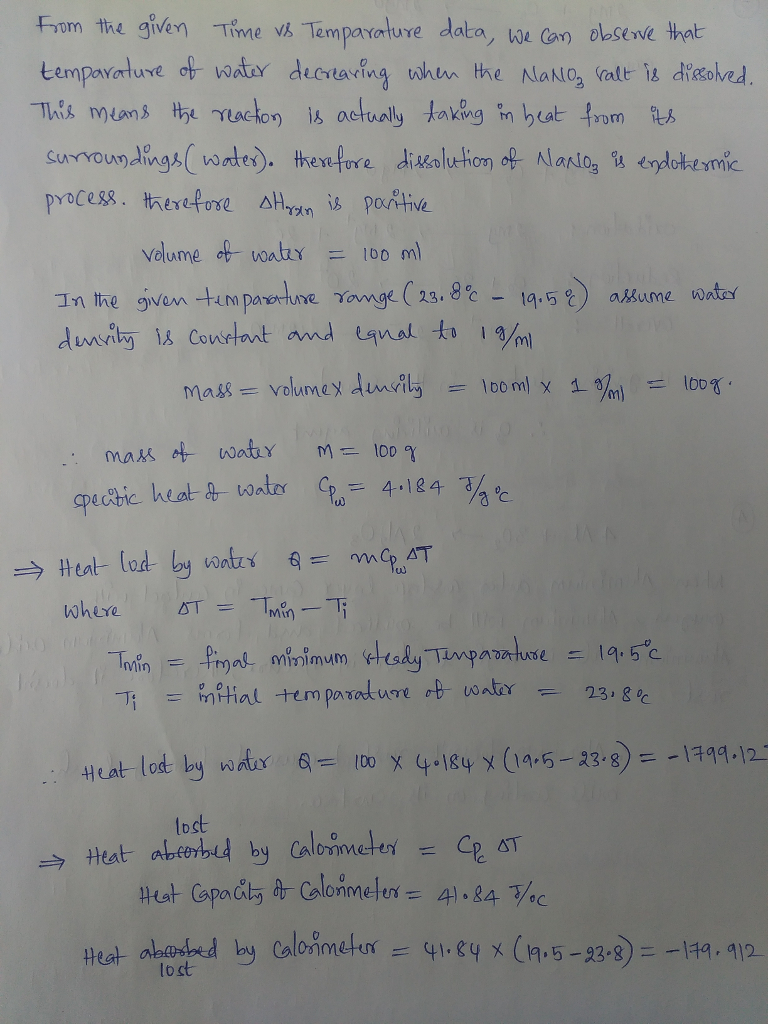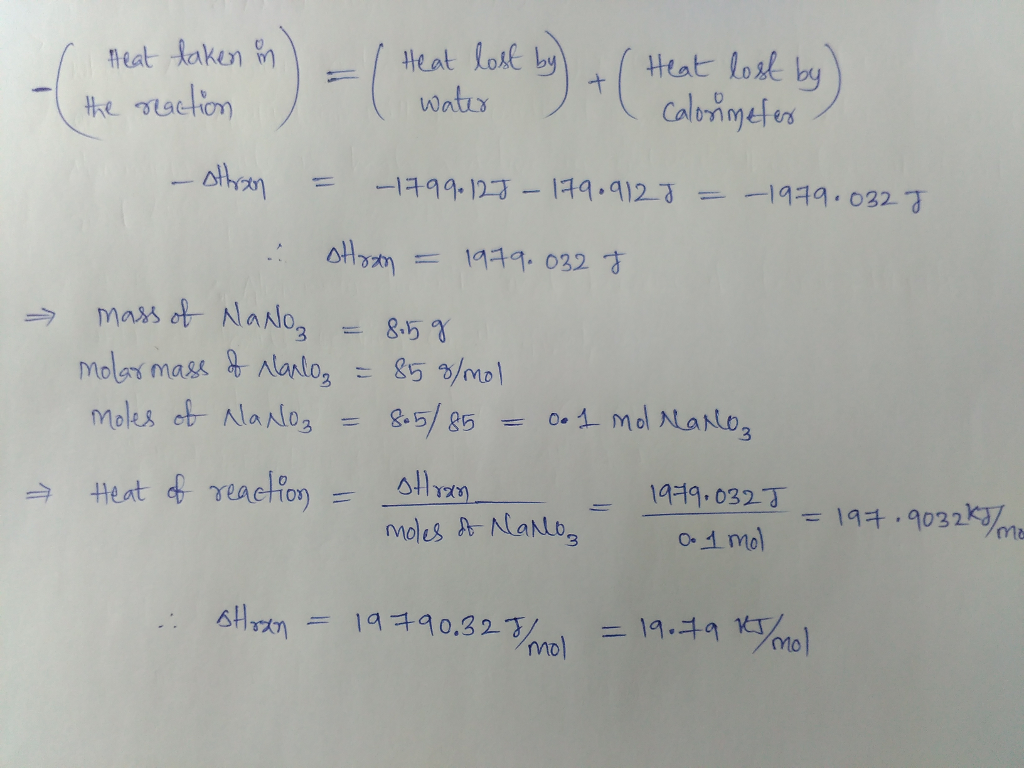# Question & Answer: In the Spontaneity experiment, the following data were collected by a student: The mass of…..In the Spontaneity experiment, the following data were collected by a student: The mass of NaNO_3 taken was 8.5 g and the volume of water taken was 100 ml. The specific heat of water is 4.184 J and the heat capacity of the calorimeter is 41.84 J. Calculate the Heat of this reaction for 1 mol of NaNO_3. (Delta H)